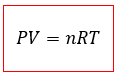# Solution: Calcium carbide reacts with water to produce acetylene gas, C 2H2.CaC2 (s) + 2 H2O (l) → Ca(OH)2 (aq) + C2H2 (g)Calculate the volume (in liters) of acetylene produced at 29°C and 684 mmHg from 0.026 mol CaC 2 and excess H2O.

###### Problem

Calcium carbide reacts with water to produce acetylene gas, C 2H2.

CaC2 (s) + 2 H2O (l) → Ca(OH)2 (aq) + C2H2 (g)

Calculate the volume (in liters) of acetylene produced at 29°C and 684 mmHg from 0.026 mol CaC 2 and excess H2O.

###### Solution

For this problem, we’re asked to calculate the volume (in liters) of acetylene (C2H2) produced at 29 °C with a pressure of 684 mmHg

The reaction occurs between excess water and 0.026 mol CaC2.

First, remember the ideal gas equationWhere,

P = Pressure (in Atm)

V = Volume (in Liters)

n = number of moles

R = Ideal gas constant (of value 0.08206 atm*L/mol*K)

T = Temperature (in K)View Complete Written Solution